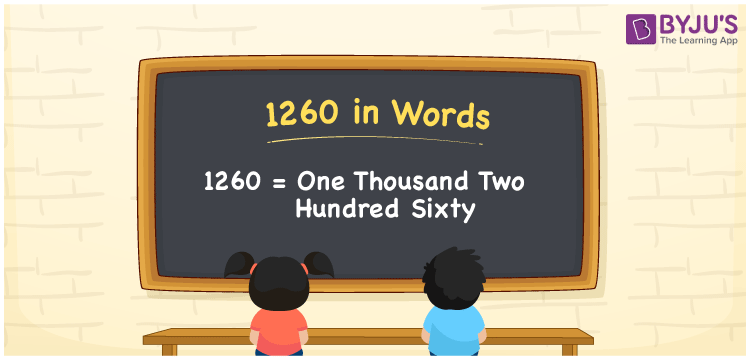# 1260 in Words

The number 1260 in words is represented as “One Thousand Two Hundred Sixty”. As we know, the number 1260 is a cardinal number as it expresses the number of objects equivalent to one thousand two hundred sixty. For example, “I have Rs. One thousand two hundred sixty rupees in my wallet”. The conversion of the number 1260 in words is done with the help of the place value system. Let us have a look at the process of writing the number 1260 in words in detail.

 1260 in Words: One Thousand Two Hundred Sixty. One Thousand Two Hundred Sixty in Numerical Form: 1260.

## 1260 in English Words## How to Write 1260 in Words?

The number 1260 has four digits. The below table shows the place values of 1260 up to 4 digits.

 Thousands Hundreds Tens Ones 1 2 6 0

The expanded form of 1260 is as follows:

= 1 × Thousand + 2 × Hundred + 6 × Ten + 0 × One

= 1 × 1000 + 2 × 100 + 6 × 10 + 0 × 1

= 1000 + 200 + 60 + 0

= 1260

= One thousand two hundred sixty

Hence, 1260 in words is One thousand two hundred sixty.

1260 in words – One thousand two hundred sixty

Is 1260 an odd number? – No

Is 1260 an even number? – Yes

Is 1260 a perfect square number? – No

Is 1260 a perfect cube number? – No

Is 1260 a prime number? – No

Is 1260 a composite number? – Yes

## Frequently Asked Questions on 1260 in Words

Q1

### Write 1260 in words.

1260 in words is one thousand two hundred sixty.

Q2

### Simplify 1000 + 260, and express it in words.

Simplifying 1000 + 260, we get 1260. Hence, 1260 in words is one thousand two hundred sixty.

Q3

### Is 1260 an odd number?

No, 1260 is not an odd number.

Test your Knowledge on 1260 in Words